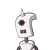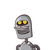# III. Answer the following 917) Find the solutions for the pair of linear equationsx+y=14x-y=418)Solve 2x-5

17) Find the solutions for the pair of linear equations
x+y=14
x-y=4
18)Solve 2x-5x+3 = 0 by using quadratic formula
19)If the area of the circle is 49rt cm, then find its perimeter
20)Show that (tanA x sin A) + cos A = secA
21) Find the sum of first twenty terms of Arithmetic series 247+12+….
22) Find the distance between the points (2,3) & (4,1)
23)Find the roots of 100x²-20x+1 = 0 by factorization
24)State the pythagoras theorem
In AABC, B = 90°, AC = 13cm, AB = 5cm. Find BC
25)Find the co-ordinates of point which divides the line segment joining
A(4,-3) and B(8,5) in the ratio of 3:2
26)The length of rectangular field is 3 times its breadth, if the area of th
metres, Find its perimeter
27)Draw a line segment of length 8cm and divide it in the ratio 5:7​

### 2 thoughts on “III. Answer the following 9<br />17) Find the solutions for the pair of linear equations<br />x+y=14<br />x-y=4<br />18)Solve 2x-5”

1.100x^2-20x+1= (10x)^2-2×10x×1+(1)^2=(10x-1)^2=(10x-1)(10x-1)

This is an identity (a-b)^2=a^2-2ab+b^2

2x^2-5x+3

a=2, b=-5, c=3

x={-b+√(b^2-4ac)}/2a=(5+1)/4=3/2

x={-b -√(b^2-4ac)}/2a=(5-1)/4=2

2.